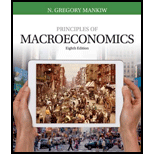# Consider an economy described by the following equations: Y = C + I + G C = 100 + 0.75( Y – T ) I = 500 – 50 r G = 125 T = 100 where Y is GDP, C is consumption, I is investment, G is government purchases, T is taxes, and r is the interest rate. If the economy were at full employment (that is, at its natural rate), GDP would be 2,000. a. Explain the meaning of each of these equations. b. What is the marginal propensity to consume in this economy? c. Suppose the central bank’s policy is to adjust the money supply to maintain the interest rate at 4 percent, so r = 4. Solve for GDP. How does it compare to the full-employment level? d. Assuming no change in monetary policy, what change in government purchases would restore full employment? e. Assuming no change in fiscal policy, what change in the interest rate would restore full employment? To find additional study resources, visit cengagebrain.com, and search for “Mankiw.”### Principles of Macroeconomics (Mind...

8th Edition
N. Gregory Mankiw
Publisher: Cengage Learning
ISBN: 9781305971509### Principles of Macroeconomics (Mind...

8th Edition
N. Gregory Mankiw
Publisher: Cengage Learning
ISBN: 9781305971509

#### Solutions

Chapter
Section
Chapter 21, Problem 11PA
Textbook Problem
4861 views

## Consider an economy described by the following equations:       Y = C + I + G       C = 100 + 0.75(Y – T)       I = 500 – 50r       G = 125       T = 100 where Y is GDP, C is consumption, I is investment, G is government purchases, T is taxes, and r is the interest rate. If the economy were at full employment (that is, at its natural rate), GDP would be 2,000. a. Explain the meaning of each of these equations. b. What is the marginal propensity to consume in this economy? c. Suppose the central bank’s policy is to adjust the money supply to maintain the interest rate at 4 percent, so r = 4. Solve for GDP. How does it compare to the full-employment level? d. Assuming no change in monetary policy, what change in government purchases would restore full employment? e. Assuming no change in fiscal policy, what change in the interest rate would restore full employment?  To find additional study resources, visit cengagebrain.com, and search for “Mankiw.”

Expert Solution

Subpart (a):

To determine

Equations describing the economy.

### Explanation of Solution

Y=C+I+G : Output is the summation of consumption, investment, and government expenditure. The equation explains the equilibrium condition for GDP or output in a closed economy.

C=100+0.75(Y-T) : The equation implies the consumption as a function of disposable income

Expert Solution

Subpart (b):

To determine

Equations describing the economy.

Expert Solution

Subpart (c):

To determine

Equations describing the economy.

Expert Solution

Subpart (d):

To determine

Equations describing the economy.

Expert Solution

Subpart (e):

To determine

Equations describing the economy.

### Want to see the full answer?

Check out a sample textbook solution.See solution

### Want to see this answer and more?

Bartleby provides explanations to thousands of textbook problems written by our experts, many with advanced degrees!

See solution

Find more solutions based on key concepts
Show solutions
Why is productivity important?

Principles of Microeconomics (MindTap Course List)

What is a loan amortization schedule, and what are some ways these schedules are used?

Fundamentals of Financial Management, Concise Edition (MindTap Course List)

What are the three authorization controls?

Accounting Information Systems

Define net exports and net capital outflow. Explain how they are related.

Principles of Macroeconomics (MindTap Course List)

ABANDONMENT OPTION The Scampini Supplies Company recently purchased a new delivery truck. The new truck costs 2...

Fundamentals of Financial Management, Concise Edition (with Thomson ONE - Business School Edition, 1 term (6 months) Printed Access Card) (MindTap Course List)

What is a remittance advice (RA)?

Pkg Acc Infor Systems MS VISIO CD